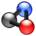# Chemical Stoichiometry

(Redirected from Actual Yield)This article is part of the ChemHelp Tutoring Wiki

In chemistry, stoichiometry deals with the quantitative relationships that exist among the reactants and products in chemical reactions. In a balanced chemical reaction, the relations among quantities of reactants and products typically form a ratio of whole numbers.

### Mass Relationships in Chemical Reactions

The ratio of stoichiometric coefficients from a balanced equation is called the stoichiometric ratio. The amounts of different reagents that participate in a chemical reaction are related through the stoichiometric coefficients in the balanced chemical equation.

### Limiting Reactants

If one reactant is in excess, the reactant that is completely consumed in a reaction is called the limiting reactant, it determines the amount of product that can form.

### Yields of Chemical Reactions

The theoretical yield is the yield that is expected (calculated) from the given quantities of reactants.

The actual yield is the yield that is produced by the actual reaction in the lab.

The percent yield is defined as $((ActualYield)/(TheoreticalYield))*100\%$.

### Combustion Analysis

In combustion analysis, a weighted sample of a compound is burned in a stream of oxygen gas.

EXAMPLE: $CH_{4}+2O_{2}->CO_{2}+2H_{2}O+heat$$C_{x}H_{y}O_{z}$and $O_{2}$-> $xCO_{2}$and $(y/2)H_{2}O$After combustion, all the carbon atoms in the sample are found in the $CO_{2}$. All the H atoms are in the $H_{2}O$Back to ChemHelp
Back to Chem154
Back to Reaction Stoichiometry and Gases